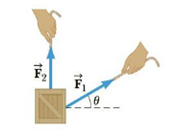Chapter 1, Problem 58P

Chapter
Section
Textbook Problem

A force F → 1 , of magnitude 6.00 units acts on an object at the origin in a direction θ = 30.0° above the positive x-axis (Fig. P1.58). A second force F → 2 of magnitude 5.00 units acts on the object in the direction of the positive y-axis. Find graphically the magnitude and direction of the resultant force F → 1 + F → 2 .Figure P1.58

To determine
The magnitude and direction of the resultant force (F1+F2) .

Explanation

Given Info: The force (F1) is having magnitude of 6units and directed along 30° above the positive x-axis.

Explanation:

The force (F2) is having magnitude of 5units and directed along the positive y-axis.

The graphical representation of the two forces and the resultant force vector is,

The second force vector is start from the head of the first vector

Still sussing out bartleby?

Check out a sample textbook solution.

See a sample solution

The Solution to Your Study Problems

Bartleby provides explanations to thousands of textbook problems written by our experts, many with advanced degrees!

Get Started